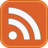# James Percival

## My Github Pages material

Last updated : 30th August 2018

# The Exner equation - Part one

Lets derive the Exner equation from first principles. Consider two surfaces, $$z=\eta(x,y)$$, denoting the boundary of a sediment bed and $$z=h(x,y)$$, denoting the free surface of the water column, as well as a curve in the x-y plane, $$\mathcal{C}(x,y)$$, bounding a cylinder, $$\Omega\times\mathbb{R}$$, in space. The total amount of sediment contained in this cylinder in this viewpoint can be written as $S = \int_{\Omega} \left[\int_{-\infty}^{\eta} (1-\phi) dz+ \int_\eta^{\eta+\Delta} c_b^*\,dz +\int_{\eta+\Delta}^h c\,dz\right]dy\,dx.$ where $$\phi(x,y,z)$$ is the bed porosity, $$c_b^*$$ is the bedload sediment and $$c$$ is the suspended sediment. Taking a time derivative, and applying the Leibnitz integral rule gives $\frac{d S}{dt} = \int_\Omega \left( (1-\phi)\frac{\partial \eta}{\partial} dy\,dx + \frac{d}{dt} \left[ \int_\eta^{\eta+\Delta} c_b^*\,dz +\int_{\eta+\Delta}^h c\,dz\right] \right) dy\,dx.$ Similarly we can consider the flux of material through the boundary, $$\mathcal{C}$$, $\frac{d S}{dt} = \oint_\mathcal{C} \left[\int_\eta^{\eta+\Delta} \left( c_b^* \mathbf{u}_b -\kappa_b \nabla c_b^*\right)\cdot \mathbf{n} \,dz + \int_{\eta+\Delta}^{h} \left( c \mathbf{u} -\kappa\nabla c \right) \cdot\mathbf{n}\,dz\right]d\ell.$ Applying the divergence theorem and making the twin ansatzes that $\Delta$ does not change with time and that changes in $$c_b$$ can be neglected, we obtain the relation $(1-\phi)\frac{\partial \eta}{\partial t} + \nabla \cdot \mathbf{q}_b + \frac{d}{dt}\int_{\eta+\Delta}^h c\,dz +\nabla\cdot \int_{\eta+\Delta}^h \left( c\mathbf{u} -\kappa \nabla c\right)\,dz=0,$ where $$\mathbf{q}_b$$ is the total flux in the bedload layer, $\mathbf{q}_b = \int_\eta^{\eta+\Delta} \left( c_b^* \mathbf{u}_b -\kappa_b \nabla c_b^* \right) \cdot \mathbf{n} \,dz.$ If we consider just the sediment in the water column, and assume that no sediment is lost through the free surface we see $\frac{d}{dt}\int_{\eta+\Delta}^h c\,dz +\nabla\cdot \int_{\eta+\Delta}^h \left( c\mathbf{u} -\kappa \nabla c \right) = F, dz$ where $$F$$ is the inward flux of material at the bottom of the free water column.

Note that we have deliberately not written $$F$$ as $$\left(c\mathbf{u}-\kappa\nabla\right)\cdot\mathbf{\hat{z}}$$, since it might arise from a different modelling process than the turbulence modelling being applied inside the free water column. Substituting this into the total sediment conservation law gives the final version of the Exner equation, $(1-\phi)\frac{\partial \eta}{\partial t} + \nabla \cdot \mathbf{q}_b = -F.$

Effectively the volume of sediment lost or gained by the bed must be balanced by a transfer of material to or from the suspended sediment, while the bedload tranport exists only to move, not hold material. It remains to define the bedload flux, $$\mathbf{q}_b$$ and the flux into the water column, as well as better describing the physics within the water column itself.

tags: scour exner